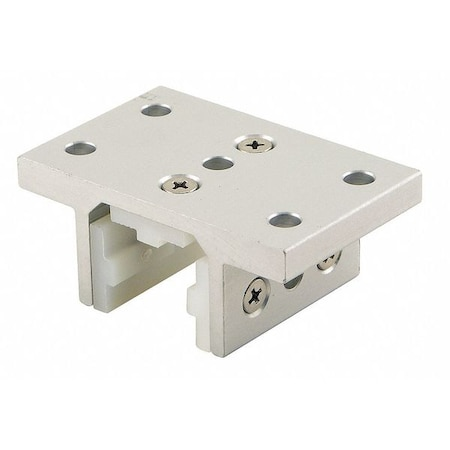80/20

# Linear Bearing, 2.937 In W, 1.875 In LTap to zoom
Hover or click to zoom
\$83.18
• Item: Linear Bearing
• Bore Dia. (In.): -
• Flange Dia. (In.): -
See full product details
This item ships free.

Total: \$83.18

## 80/20 #6425 Specifications

• Item: Linear Bearing
• Bore Dia. (In.): -
• Flange Dia. (In.): -
• Flange Dia. (mm): -
• Material: UHMWPE
• Dimension S: -
• Steady State Speed - Continuous Dry: -
• Dimension M (In.): 0.906 in
• Dimension H: -
• Dimension A1: -
• Retaining Ring: -
• Dimension E: -
• Dimension B: -
• Temp. Range (F): -
• Slot Angle (Degrees): -
• Dimension E (In.): 0.812 in
• Dimension E1 (In.): -
• Barrel Dia. (mm): -
• Flange Thickness (In.): 0.312 in
• Dynamic Load Capacity (N): -
• Mounting Hole Center (In.): 1 in
• Dimension L (In.): 1.218 in
• Mounting Surface to Shaft Center (In.): -
• Dimension A2: -
• Body Width (In.): 2.937 in
• Max. Pv: -
• Dimension F1: -
• Steady State Speed - Intermittent Dry: -
• Type: Double Flange
• Overall Width (mm): -
• Dimension Dp: -
• Dimension H (In.): 0.656 in
• Dimension A: -
• Dimension O: -
• Dimension E2: -
• Dimension P: 0.438 in
• Static Load Capacity (N): -
• Mounting Bolt (In.): 1/4-20
• Dimension Z: -
• Mounting Surface to Shaft Center (mm): -
• For Use With: 10 Series
• Slot Width (mm): -
• Dimension I: -
• Dimension F: -
• Dimension N Dia. (In.): -
• Overall Height (mm): -
• Dimension D (In.): 0.406 in
• Liner: -
• Dimension R: -
• Mounting Hole (In.): 0.257 in
• Dimension J: -
• Dimension E1: -
• Dimension N2: -
• Dimension D: -
• Overall Width (In.): 2.937 in
• Dimension C: -
• Body Width (mm): -
• Dimension G1 (In.): -
• Mounting Hole Dia. (In.): 0.257 in
• Dimension V (In.): -
• Bore Dia. (mm): -
• Dimension A (In.): 1 in
• Dimension G: -
• Dimension H1: -
• Color: Silver
• Overall Length (mm): -
• Dimension Y: -
• Lubrication: Dry
• Dynamic Load Capacity (Lb.): -
• Bolt Size: 1/4-20
• Dimension B (In.): 0.312 in
• Dimension d: -
• Dimension G (In.): 2.937 in
• Width: 2.937
• Dimension G1: -
• Accelerated Speed: -
• Static Load Capacity (Lb.): -
• Dimension F (In.): 0.312 in
• Dimension N: -
• Overall Length (In.): 1.875 in
• Self-Alignment: -
• Flange Thickness (mm): -
• Dimension A1 (In.): -
• Dimension H1 (In.): -
• Includes: -
• Mounting Hole Center (mm): -
• Overall Height (In.): 1.218 in
• Dimension C (In.): 1.625 in
• Dimension N2 (In.): -
• Dimension F1 (In.): -
• Slot Width (In.): 0.255 in
• Height: 1.218
• Seal: -
• Locking Type: Optional
• Mounting Hole Dia. (mm): -
• Finish: Clear Anodize
• Dimension X: -
• Agency Compliance: -
• Dimension A2 (In.): -
• Dimension K: 0.812 in
• Dimension N3: -
• Dimension V: -
• Barrel Dia. (In.): -
• Dimension S (In.): -
• Shaft Dia. (In.): -
• Product Type: Linear Bearing
• For Number of Slots: 3
• Overall LxW: 1 7/8 in x 2 15/16 in
• Overall Height: 1 7/32 in
• Overall Width: 2 15/16 in
• Overall LxWxH: 1 7/8 in x 2 15/16 in x 1 7/32 in
• Flange Thickness: 5/16 in
• Overall Length: 1 7/8 in
• Bearing Profile: Double Flanged
• Mounting Hole Diameter: 17/64 in
• Compatible Product Type: 10 Series
• Series: 10 Series
• System of Measurement: Inch